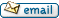•http://facebook.com/
•https://www.google.com/accounts/o8/id
•https://me.yahoo.com

# position uniform

Position of an object moving uniformly
Controller: CodeCogsPrivate project under development, to help contact the author:C++
Excel

## Overview

This module computes the position of an object moving uniformly (constant speed or constant acceleration).

### Authors

Lucian Bentea (July 2007)

## Position Const Velocity

 doubleposition_const_velocity( double t double v double x0 = 0 double t0 = 0 )[inline]
This function determines the position of an object at time after moving uniformly at constant velocity for a certain period of time. The formula relating the above quantities is where is the initial position of the object at time .

### Example 1

#include <codecogs/physics/kinematics/position_uniform.h>
#include <iostream>

int main()
{
// the constant velocity and the current time
double v = 5.7, t = 12;

std::cout << std::endl;
std::cout << "Velocity = " << v << " m/s";
std::cout << std::endl;
std::cout << "    Time = " << t << " s";
std::cout << std::endl << std::endl;

// compute the current position
// assuming the initial position and time are null

std::cout << "Position = " <<
Physics::Kinematics::position_const_velocity(t, v);
std::cout << " m" << std::endl;

return 0;
}

### Output

Velocity = 5.7 m/s
Time = 12 s

Position = 68.4 m

### Parameters

 t the current time (seconds) v the value of the constant velocity (meters per second) x0 Default value = 0 t0 Default value = 0

### Returns

the position of the object after moving uniformly at constant velocity for t seconds (meters)
##### Source Code

This module is private, for owner's use only.

Not a member, then Register with CodeCogs. Already a Member, then Login.

## Position Const Acceleration

 doubleposition_const_acceleration( double t double a double v0 double x0 = 0 double t0 = 0 )[inline]
This function determines the position of an object at time after moving uniformly at constant acceleration for a certain period of time, with initial velocity given by . The formula relating the above quantities is where is the initial position of the object at time .

### Example 2

#include <codecogs/physics/kinematics/position_uniform.h>
#include <iostream>

int main()
{
// the constant velocity and the current time
double a = 0.7, v0 = 4.33, t = 9.8;

std::cout << std::endl;
std::cout << "  Acceleration = " << a << " m/s^2";
std::cout << std::endl;
std::cout << "Init. velocity = " << v0 << " m/s";
std::cout << std::endl;
std::cout << "          Time = " << t << " s";
std::cout << std::endl << std::endl;

// compute the current position
// assuming the initial position and time are null

std::cout << "      Position = " <<
Physics::Kinematics::position_const_acceleration(t, a, v0);
std::cout << " m" << std::endl;

return 0;
}

### Output

Acceleration = 0.7 m/s^2
Init. velocity = 4.33 m/s
Time = 9.8 s

Position = 76.048 m

### Parameters

 t the current time (seconds) a the value of the constant acceleration (meters per sq. second) v0 the initial velocity of the object at time t0 (meters per second) x0 Default value = 0 t0 Default value = 0

### Returns

the position of the object after moving uniformly at constant acceleration for t seconds (meters)
##### Source Code

This module is private, for owner's use only.

Not a member, then Register with CodeCogs. Already a Member, then Login.